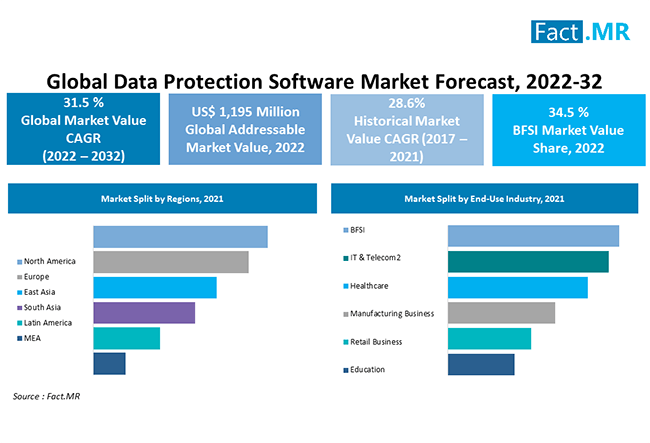# Swish Activation Function

• Swish is a smooth continuous function, unlike ReLU which is a piecewise linear function.
• Swish allows a small number of negative weights to be propagated through, while ReLU thresholds all negative weights to zero. This is an extremely important property and is crucial in the success of non-monotonic smooth activation functions, like that of Swish, when used in increasingly deep neural networks.
• In Swish, the trainable parameter allows to better tune the activation function to maximize information propagation and push for smoother gradients, which makes the landscape easier to optimize, thus generalizing better and faster.
• Leaky ReLU, where f(x) = x if x ≥ 0, and ax if x < 0, where a = 0.01. This allows for a small amount of information to flow when x < 0, and is considered to be an improvement over ReLU.
• Parametric ReLU is the same as Leaky ReLU, but a is a learnable parameter, initialized to 0.25.
• Softplus, defined by f(x) = log(1 + exp(x)), is a smooth function with properties like Swish, but is strictly positive and monotonic.
• Exponential Linear Unit (ELU), defined by f(x) = x if x ≥ 0 and a(exp(x) — 1) if x < 0 where a = 1.
• Scaled Exponential Linear Unit (SeLU), identical to ELU but with the output multiplied by a value s.
Previous Article## Multiple Time Series Forecasting in Python

Next Article## Attributes of Cybersecurity Promotes The Growth of Advanced Data Protection Software| Latest Report - Fact.MR Industry Research Blog

##### Related Posts## WhatsApp makes it easier for friends and family to join a call with just a tap

What you need to know WhatsApp has introduced a new calling feature for its mobile app. Call Links…## 2D Vector local to global space without trig/sqrt

Per this answer to this question, a cheap way (i.e., without trig/sqrt) to convert from global to local…